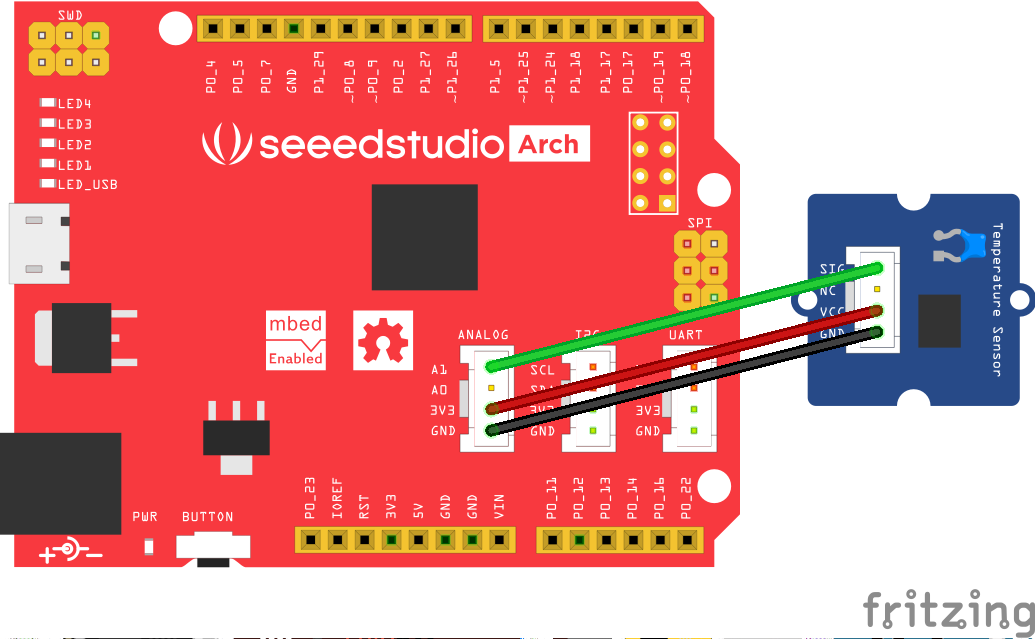### Seeed

A hardware innovation platform for makers to grow inspirations into differentiating products.

## Analog Temperature Sensor

Grove - Temperature Sensor can convert temperature value to voltage value, which can be read by the Arch board. In this example, you'll make a thermometer. The temperature is displayed as LED blinks. LED4 blinks corresponding to tens place of temperature value(in deg C) and LED1 blinks according to units place.

### Hardware¶

• Arch Board
• Grove - Temperature Sensor### Software¶

Import the following code to mbed online compiler

```#include "mbed.h"

AnalogIn thermistor(A1);   /* Temperature sensor connected to Analog Grove connector */

DigitalOut tensplaceLED(LED4);  /* This led blinks as per tens place of temperature value(in deg C) */
DigitalOut unitsplaceLED(LED1); /* This led blinks as per units place of temperature value(in deg C) */

int main()
{
unsigned int a, beta = 3975, units, tens;
float temperature, resistance;

while(1) {

/* Calculate the resistance of the thermistor from analog votage read. */
resistance= (float) 10000.0 * ((65536.0 / a) - 1.0);

/* Convert the resistance to temperature using Steinhart's Hart equation */
temperature=(1/((log(resistance/10000.0)/beta) + (1.0/298.15)))-273.15;

units = (int) temperature % 10;
tens  = (int) temperature / 10;

for(int i=0; i< tens; i++)
{
tensplaceLED = 1;
wait(.200);
tensplaceLED = 0;
wait(.200);
}

for(int i=0; i< units; i++)
{
unitsplaceLED = 1;
wait(.200);
unitsplaceLED = 0;
wait(.200);
}

wait(0.5);
}
}
```